Shipra Saxena — April 5, 2021

## Objective

• The activation function is one of the building blocks on Neural Network
• Understand how the Softmax activation works in a multiclass classification problem

## Introduction

The activation function is an integral part of a neural network. Without an activation function, a neural network is a simple linear regression model. This means the activation function gives non-linearity to the neural network.

Note: If you are more interested in learning concepts in an Audio-Visual format, We have this entire article explained in the video below. If not, you may continue reading.

If you want to dig deeper, I will recommend you to go through the following article.

Fundamentals of Deep Learning – Activation Functions and When to Use Them?

In this article, we will discuss the SoftMax activation function. It is popularly used for multiclass classification problems. Let’s first understand the neural network architecture for a multi-class classification problem and also why other activation functions can not be used in this case.

## Example

Suppose, we have the following dataset and for every observation, we have five features from FeatureX1 to FeatureX5 and the target variable has three classes.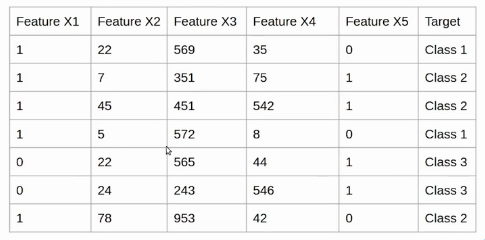Now let’s create a simple neural network for this problem. Here, we have an Input layer with five neurons as we have five features in the dataset. Next, we have one hidden layer which has four neurons. Each of these neurons uses inputs, weights, and biases here to calculate a value which is represented as Zij here.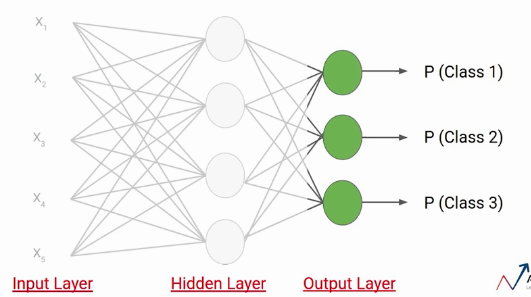For example, the first neuron of the first layer is represented as Z11 Similarly the second neuron of the first layer is represented as Z12, and so on.

Over these values, we apply the activation function. Let’s say a tanh activation function and send the values or result to the output layer.

The number of neurons in the output layer depends on the number of classes in the dataset. Since we have three classes in the dataset we will have three neurons in the output layer. Each of these neurons will give the probability of individual classes. This means the first neuron will give you the probability that the data point belongs to class 1. Similarly, the second neuron will give you the probability that the data point belongs to class 2 and so on.

## Why Not Sigmoid?

Suppose we calculate the Z value using weights and biases of this layer and apply the sigmoid activation function over these values. We know that the sigmoid activation function gives the value between 0 and 1. suppose these are the values we get as output.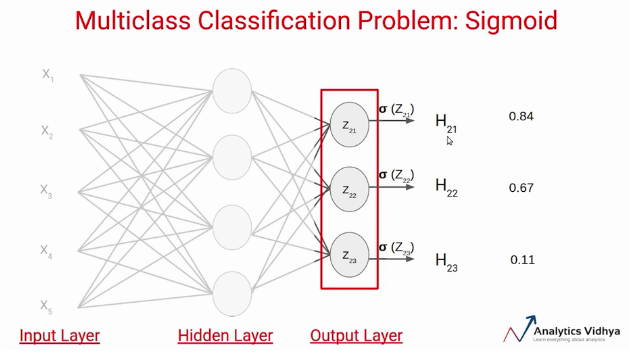There are two problems in this case-

First, if we apply a thresh-hold of say 0.5, this network says the input data point belongs to two classes. Secondly, these probability values are independent of each other. That means the probability that the data point belongs to class 1 does not take into account the probability of the other two classes.

This is the reason the sigmoid activation function is not preferred in multi-class classification problems.

## Softmax Activation

Instead of using sigmoid, we will use the Softmax activation function in the output layer in the above example. The Softmax activation function calculates the relative probabilities. That means it uses the value of Z21, Z22, Z23 to determine the final probability value.

Let’s see how the softmax activation function actually works. Similar to the sigmoid activation function the SoftMax function returns the probability of each class. Here is the equation for the SoftMax activation function.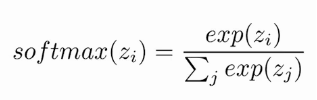Here, the Z represents the values from the neurons of the output layer. The exponential acts as the non-linear function. Later these values are divided by the sum of exponential values in order to normalize and then convert them into probabilities.

Note that, when the number of classes is two, it becomes the same as the sigmoid activation function. In other words, sigmoid is simply a variant of the Softmax function. If you want to learn more about this concept, refer to this link.

Let’s understand with a simple example how the softmax works, We have the following neural network.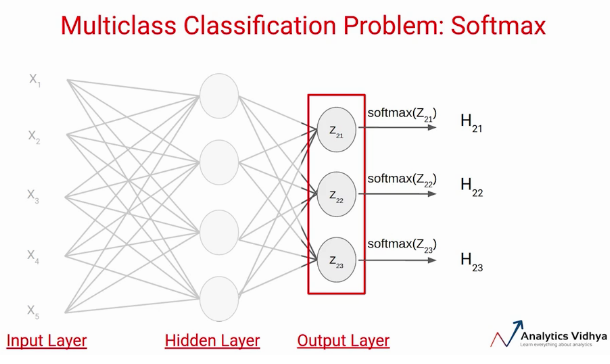Suppose the value of Z21, Z22, Z23 comes out to be 2.33, -1.46, and 0.56 respectively. Now the SoftMax activation function is applied to each of these neurons and the following values are generated.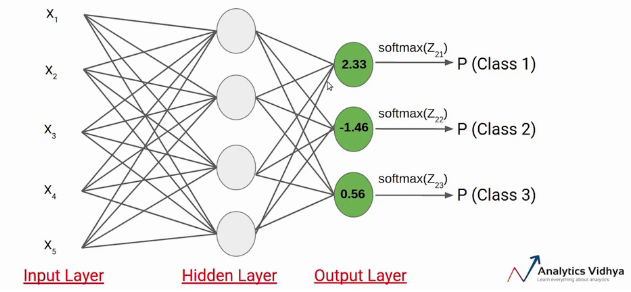These are the probability values that a data point belonging to the respective classes. Note that, the sum of the probabilities, in this case, is equal to 1.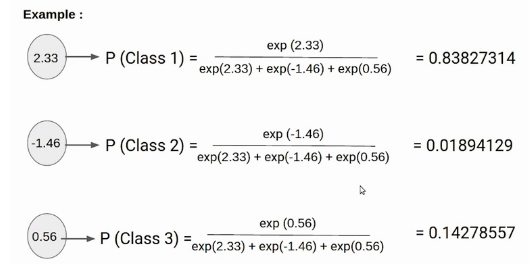In this case it clear that the input belongs to class 1. So if the probability of any of ￼these classes is changed, the probability value of the first class would also change.

## End Notes

This is all about the SoftMax activation function in this article. Here we saw why we should not use activation functions like sigmoid or tanh in the multiclass classification problems and also how softmax function works through an example.

If you are looking to kick start your Data Science Journey and want every topic under one roof, your search stops here. Check out Analytics Vidhya’s Certified AI & ML BlackBelt Plus Program

If you have any queries let me know in the comments below!